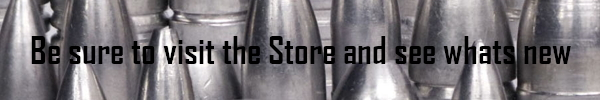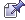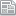###Author Topic: Whats all the information?  (Read 4009 times)

#### SwedeNelson##### Whats all the information?
« on: March 24, 2017, 10:48:16 PM »
What is all the information for on our drawing?

What is "Sectional Density" and what is it used for?

•   Sectional Density is a value that relates the ratio of the density, or weight, to the cross-sectional area of a bullet.

•   Sectional Density is used in the calculation of a Ballistic Coefficient.
Ballistic Coefficient = Sectional Density divided by the bullet Form Factor.
The Weight of a bullet, in pounds, is divided by the diameter squared of the bullet.
Weight in grains = G
Weight in pounds = P (There are 7,000 grains in one pound)
Diameter in inches = D
Sectional Density = SD
P = G ÷ 7000
SD = P ÷ D ÷ D
A 250 gr. bullet of 0.454 diameter has a Sectional Density value of.
SD = ( 250 ÷ 7000 ) ÷ .454 ÷ .454 = 0.1732

What is "Form Factor" and what is it used for?

•   The "Form Factor" or Coefficient of Form is a mathematically derived number that compares the profile of a bullet to the profile a "Standard Projectile".

•   The Form Factor is used to calculate the Ballistic Coefficient of a bullet with a specific nose profile.
Ballistic Coefficient = Sectional Density divided by the bullet Form Factor.
While the form factor of a bullet is best determined by experimental firing tests, a function exists that closely ESTIMATES the G1 Drag Table form factor for pointed jacketed bullets using the shape of the bullet nose that is presented toward the ballistic wind.
The nose shape of a tangential ogive bullet may be defined and given a value by determining the Ogive Radius of the nose curve.
The term Ogive Radius is used to describe the curved portion of the bullet nose.  It is the radius of the curve of the nose defined in caliber lengths.
Three measurements are required to define the curve radius length.
1. The length of the curved portion of the nose = NL
2. The diameter of the nose where the curved portion begins = D
3. The diameter of the tip or me plate, whether rounded or flat, = T
The length, L, in inches,  of the curve radius may be calculated by the following function.
L = ( NL × NL ) ÷  ( D - T ) + ( D - T) ÷ 4
Using the value of the Ogive Radius in a function will return a jacketed, pointed bullet form factor value for the G1 Resistance Table as follows:
F =  G1 Form Factor.
R = Ogive radius in nose diameters.
R = L ÷ D  (Ogive Radius, in inches, divided by nose diameter, in inches)
The basic function to determine the form factor of tangential ogive, sharp pointed, jacketed bullets is:
F =  2 ÷ R × Square Root of [   ( 4 × R - 1 ) ÷  7  ]
Using a highly modified version of this function, Form Factors and the resulting Ballistic Coefficients for cast bullets can be closely ESTIMATED.

What is the “Estimated B. C.”?

•   B.C. is the abbreviation of "Ballistic Coefficient"

•   Basically, B.C. is a measure of how streamlined a bullet is; that is, how well it cuts through the air.

•   Essentially B.C. is a measure of "air drag deceleration" of the bullet during it's trajectory.

•   Mathematically,  B.C. is the ratio of a bullet's "Sectional Density" to it's "Coefficient of Form".
What is "Estimated BC" used for?

•   The Ballistic Coefficient is used to calculate  bullet trajectory factors, such as time of flight, bullet drop and remaining velocity and energy at various distances along the trajectory path.
The ballistic coefficient is a number that relates the air-drag deceleration of an actual bullet to the air-drag deceleration of a standard bullet. Extensive testing was performed to determine and record these air-drag or retardation values throughout a useful velocity range. These retardation values are then listed in a Retardation Table , or Drag Table (usually in 10 feet per second intervals ).
The most commonly used table is referenced as the G1 table and was tabulated using the retardation values of a projectile weighing l pound, with a diameter of 1 inch and a nose curvature having a radius of 2 calibers ( or two inches ).
A smaller diameter bullet of lesser weight will posses lesser inertia than that of the standard bullet and will not pierce the air as easily as the standard bullet. The result of this condition is that a smaller bullet will posses larger retardation values spanning the useful velocity range than the standard bullet.
If the actual bullet produces retardation values that are double those which the standard bullet produces, then the actual bullet may be compared to the standard bullet with a coefficient number obtained by dividing the retardation rate of the standard bullet by the retardation rate of the actual bullet.
The result of this division is a G1 ballistic coefficient of 0.500 for the actual bullet. [Standard Bullet Retardation] / [Actual Bullet Retardation] = Ballistic Coefficient of the Standard bullet.
This ballistic coefficient is valid only for the G1 Retardation Table and would have no useful application with any other retardation table.
Calculating a ballistic coefficient from the physical description of a bullet requires two values, the Sectional Density of the bullet, which may be directly calculated, and a Form Factor value which may be estimated using the process described in the Form Factor topic.
After these values are determined, the Ballistic Coefficient is easily calculated.
Ballistic Coefficient = Sectional Density divided by the bullet Form Factor.
BC = Ballistic Coefficient
SD = Sectional Density
FF = Form Factor
BC = SD ÷ FF

Other standard bullet profiles have been tested and the drag deceleration values tabulated into drag  or retardation tables relating to each profile.
G2 (Aberdeen J projectile), long nose, long boat tail bullets
G5 (short 7.5° boat-tail, 6.19 calibers long tangent ogive)
G6 (flat base, 6 calibers long secant ogive)
G7 (long 7.5° boat-tail, 10 calibers tangent ogive, preferred by some manufacturers for very-low-drag bullets)
G8 (flat base, 10 calibers long secant ogive)
GL (blunt lead nose) Essentially a modification of the G1 Table allowing for soft lead tip deformation at velocities above 1300 fps.

What is "C.G."  and what is it used for?

•   The "Center of Gravity", or Center of Mass, of a bullet is the point at which all the weight of the bullet seems to be concentrated. That is, there is as much mass, or  weight, behind the center of gravity as there is ahead of the center.

•   The relative positions along the bullet axis and distance between the Center of Gravity and Center of Pressure may be used, to evaluate the "Destabilizing Effect" of the Ballistic Wind upon bullet in flight..
The Center of Gravity may be physically determined by balancing a bullet on a knife edge.
The Center of Gravity and the Center of Pressure on a bullet such as a double ended wad-cutter would be at the same point, exactly at the center point of the axis of the bullet. However, if the bullet is composed of different profiles on the nose and base, then the CG and CP would be at different points along the axis of the bullet.

What is "C.P." and what is it used for?

•   The "Center of Pressure", or Profile Centroid, of a bullet is the point at which all the air pressure forces on the bullet seem to be concentrated. That is, there is as much air pressure force on the bullet behind the center of pressure as there are ahead of the center.

•   The relative positions along the bullet axis and distance between the Center of Pressure and Center of Gravity may be used, to evaluate the "Destabilizing Effect" of the Ballistic Wind upon bullet in flight.
The Center of Pressure of a bullet may be physically determined by balancing a a scaled up cardboard cutout of the Profile of the bullet on a knife edge.
The Center of Gravity and the Center of Pressure on a bullet such as a double ended wad-cutter would be at the same point, exactly at the center point of the axis of the bullet. However, if the bullet is composed of different profiles on the nose and base, then the C.G. and C.P. would be at different points along the axis of the bullet.

What is the "Destabilizing Effect"?

When a spin stabilized bullet travels along the trajectory path, the gyroscopic effect of the spin attempts to maintain a bullet in the initial nose-up position which was determined by the required sighting elevation.
This slight nose-up attitude presents the PROFILE of the bullet to the pressure of the Ballistic Wind of the trajectory. The base of an average bullet has a larger profile than the nose which applies more Ballistic Wind pressure to the bullet base and tends to lift the base of the bullet more into alignment with the path of the trajectory. However the downward angle of the trajectory is constantly being increased by gravity so the spin stabilized bullet never quite catches up and is always traveling in a slightly nose-up attitude in relation to the trajectory path.
Depending upon the design of the bullet, the Center of Pressure is usually closer to the nose of the bullet than the Center of Gravity.
A rotating bullet is seldom, if ever, perfectly balanced and therefore gyrates and precesses around the Center of Gravity of the bullet as it travels along the trajectory.
If the Center of Pressure is not at the same point as the Center of Gravity, the pressure of the Ballistic Wind acting upon the Center of Pressure will tend to increase the unbalanced condition of the bullet.
The distance from the Center of Pressure to the Center of Gravity may be considered as a lever to unbalance the bullet. The larger the distance between the two, the longer the lever, resulting in a larger destabilization force.
So, the generally accepted theory is that, the closer the Center of Pressure to the Center of Gravity, the more potential for a stable bullet.
The "balance point" of a bullet would be the center of mass or center of gravity ( CG )
The Center of Gravity may be calculated by finding the average of the distances from the bullet base of the Center of Gravity of each of the shanks, bands, grooves, bore rider, nose, etc. that make up the design of that particular bullet.
The Center of Gravity may be physically determined by balancing a bullet on a knife edge.
The Center of Pressure may be calculated by the determining the balance point of the entire PROFILE of the bullet and can be defined as the average of the distances from the bullet base of the Area Center of each of the various section profiles of the bullet.
The Center of Pressure of a bullet may be physically determined by balancing a a scaled up cardboard cutout of the Bullet Profile of the bullet on a knife edge.
A suggested procedure to move the center of pressure closer to the center of gravity on an existing design would be to produce that design in a hollow base version with the hollow base cavity volume calculated to move the Center of Gravity forward to coincide with the existing Center of Pressure.

« Last Edit: May 02, 2017, 11:54:37 PM by SwedeNelson »

There are any comments for this topic. Do you want to be the first?